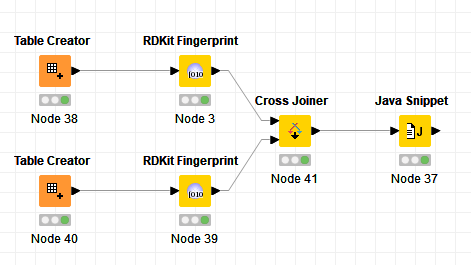# Similarity search with user defined coefficients

Dear all,

I am relatively new to KNIME and are trying to do similarity searches, between a dataset containing fingerprints and a dataset containing reference fingerprints.
However, for the similarity search I want to make use of less common similarity coefficients that are not incorporated within, for instance, the similarity search node:

Simple matching: s= (a+d)/(a+b+c+d)
Sokal-Sneath: s= 1/4 [a/(a+b)+a/(a+c)+d/(b+d)+d/(c+d)]

In which a, b, c and d are:

• a = number of bits set in both fingerprints (=1)
• d = number of bits not set in both fingerprints (=0)
• b = number of bits set in fingerprint but not in reference fingerprint
• c = number of bits not set in fingerprint but set in reference fingerprint

I thought that the use of the similarity search node with a user provided distance measure (using the Java distance node) would be the solution. However, I cannot figure out how to make this work.
Any help or suggestions would be highly appreciated.

Kind regards,
Pim

Hi Pim,

this is going to be challenging. The node was made having in mind to calculate similarities on strings.

There is no native support for bit vectors in the node. However, just tested, dense bit vectors (an option you can set in the “Create Bit Vector” node) are passed in as a string of bits (“011000111…”). Sparse bit vectors are not (and you are out of luck).

I think even with the “000111” representation you will have a hard time calculating a, b, c, and d as the nodes doesn’t offer methods to do that right away (and the entire expression needs to fit int a single statement, no code blocks).

Unfortunately, I think, the node won’t help you solve that problem. Maybe consider to write a custom node to do it?

Bernd

After writing about using Vernalis Knime nodes, I deleted that part as I thought it might be a lot easier to just iterate the Bit Strings (assuming both datasets have same length). You can simply iterate the bitstrings in a index-based for loop to calculate a,b,c and d. Albeit not sure how performant that will be on large datasets.

``````double a = 0;
double b = 0;
double c = 0;
double d = 0;
for (int i = 0; i < c_Fingerprint.length(); i++){
char bit = c_Fingerprint.charAt(i);
char refBit = c_Fingerprint1.charAt(i);
//compare bits and manage counts
if (bit == 49 && refBit == 49) { // 49 is char code for 1 and 48 for 0
a++;
} else if (bit == 48 && refBit == 48) {
d++;
} else {
if (bit == 49) {
b++;
} else {
c++;
}
}
}

out_Simplematching = (a+d)/(a+b+c+d);
out_SokalSneath = (2*a + 2*b) / (2*a + b + c + 2*d );
``````

I advise you to really asses my logic. Not sure it’s 100% correct but should get the idea across. Also verify the sokal-sneath formula. I wasn’t sure what you meant by the braces and found the formula I used via google.

You can use the code in a Java snippet with an input table that already contains all the pairs to compare, eg. generated via cross-joiner:Note that of course the cross-joining can lead to a lot of rows very quickly. So that will only really work reasonably fast if the data sets are rather small (but same would apply to similarity search node as well).

Custom Distance.knwf (13.4 KB)

3 Likes